Question

Sections 12.1-12.2 For Problems 12.1 through 12.17 use the force method (method of con sistent distortions)...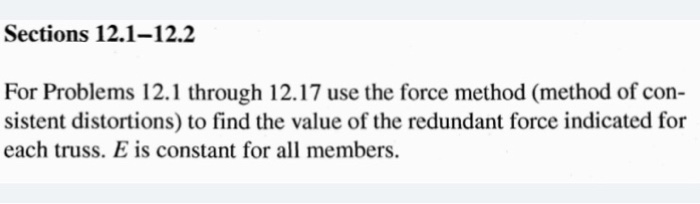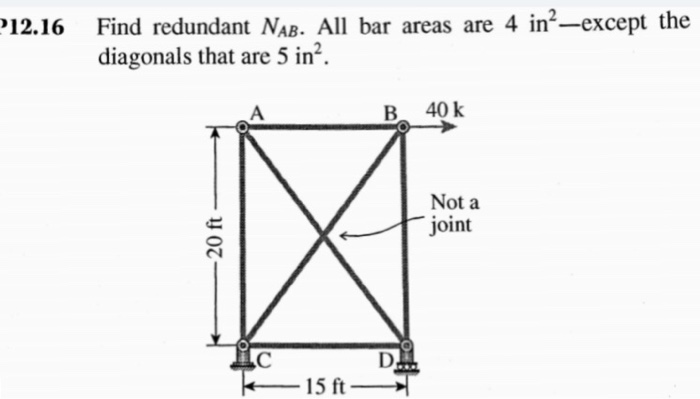Sections 12.1-12.2 For Problems 12.1 through 12.17 use the force method (method of con sistent distortions) to find the value of the redundant force indicated for each truss. E is constant for all members.

Solution:-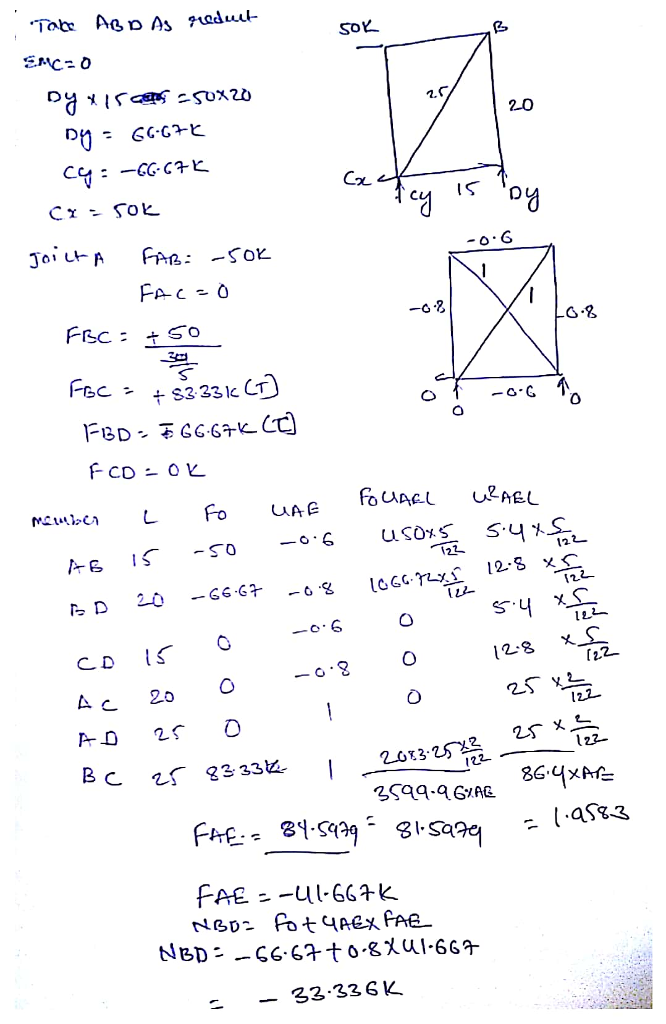Earn Coins

Coins can be redeemed for fabulous gifts.

Similar Homework Help Questions
• Use the force method (method of consistent distortions) to find the value of the redundant force ...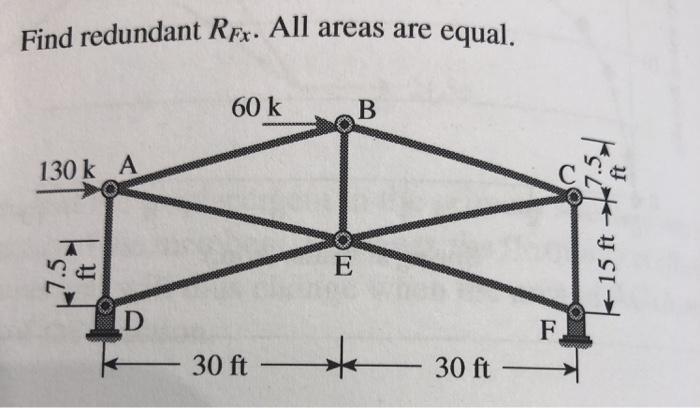Use the force method (method of consistent distortions) to find the value of the redundant force indicated in each truss. E is constant for all members.

• For Problems 9.7 through 9.29, use the virtual work method using con- ventional integration and only...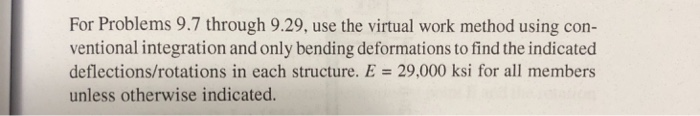For Problems 9.7 through 9.29, use the virtual work method using con- ventional integration and only bending deformations to find the indicated deflections/rotations in each structure. E = 29,000 ksi for all members unless otherwise indicated.

• Part B: Numerical Problems (15 points each) 1. For the truss loaded as shown, (a) use the Method of Joints to determine the force in member CF, and then (b) use the Method of Sections to determine...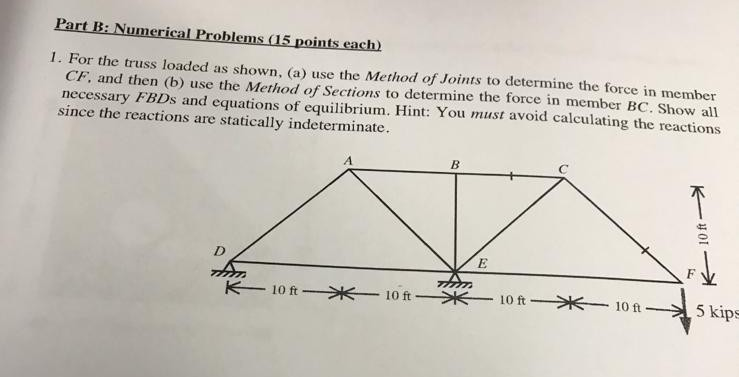Part B: Numerical Problems (15 points each) 1. For the truss loaded as shown, (a) use the Method of Joints to determine the force in member CF, and then (b) use the Method of Sections to determine the force in member BC. Show all necessary FBDs and equations of equilibrium. Hint: You must avoid calculating the reactions since the reactions are statically indeterminate. 5 kips Part B: Numerical Problems (15 points each) 1. For the truss loaded as shown, (a)...

• 311. A truss is shown. Use the method of sections to find the force in members...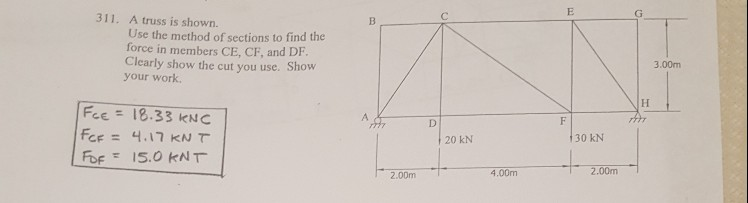311. A truss is shown. Use the method of sections to find the force in members CE, CF, and DF. Clearly show the cut you use. Show your work. 3.00m Fee = 18.33 kNC FCF = 4.17 KN T 20 kN 30 kN 2.00m 4.00m 2.00m

• t The Method of Sections 1 of 5 Part A Learning Goal: To apply the method...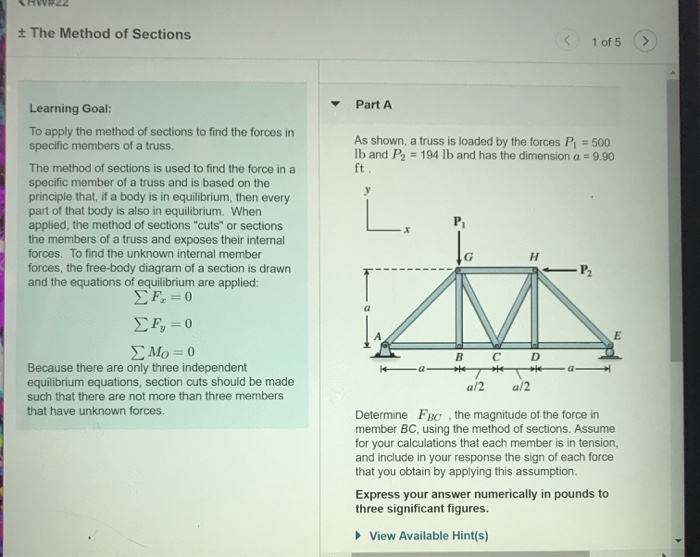t The Method of Sections 1 of 5 Part A Learning Goal: To apply the method of sections to find the forces in specific members of a truss. As shown, a truss is loaded by the forces P 500 lb and P2 194 lb and has the dimension a 9.90 ft The method of sections is used to find the force in a specific member of a truss and is based on the principle that, if a body is in...

• I need to determine the force of a truss using the Method of Sections

I need to determine the Force through the Method of Sections (Tension or compression) in members EG, CG and BC of the truss. I do have a pic of it, but do not know howto attach it. Can someone help?

• ***PLEASE SOLVE BY METHOD OF SECTIONS IF POSSIBLE***​​​​​​​​​​​​​​***PLEASE SOLVE BY METHOD OF SECTIONS IF POSSIBLE*** 3....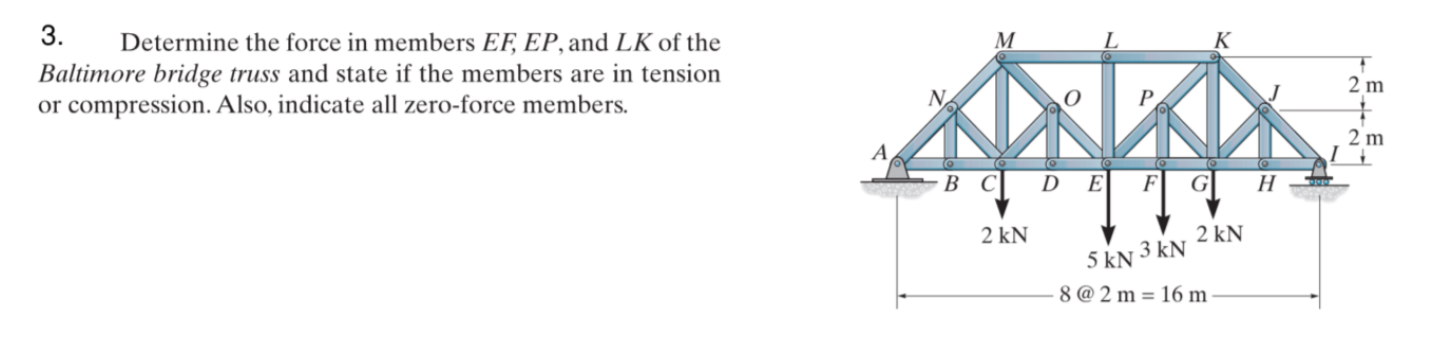***PLEASE SOLVE BY METHOD OF SECTIONS IF POSSIBLE***​​​​​​​​​​​​​​***PLEASE SOLVE BY METHOD OF SECTIONS IF POSSIBLE*** 3. Determine the force in members EF, EP, and LK of the Baltimore bridge truss and state if the members are in tension or compression. Also, indicate all zero-force members. 2 m PR 2 m ООО D E F G H 2 kN 2 KN 5 kN 3 kN -8 @ 2 m = 16 m

• Use the Method of Sections to find the force in members BD, BE, & CE below....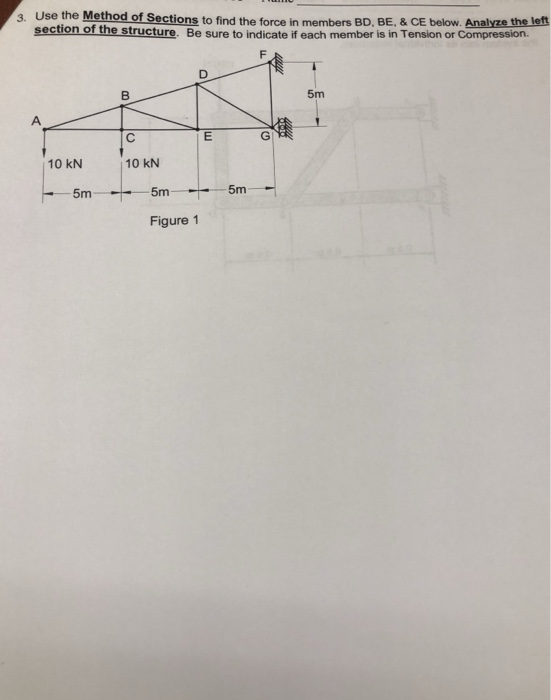Use the Method of Sections to find the force in members BD, BE, & CE below. Analyze the left section of the structure. Be sure to indicate if each member is in Tension or Compression. 5m 10 kN 10 kN 5m 5m Figure 1

• a) Identify all zero force members b) Use the METHOD OF SECTIONS to determine the member...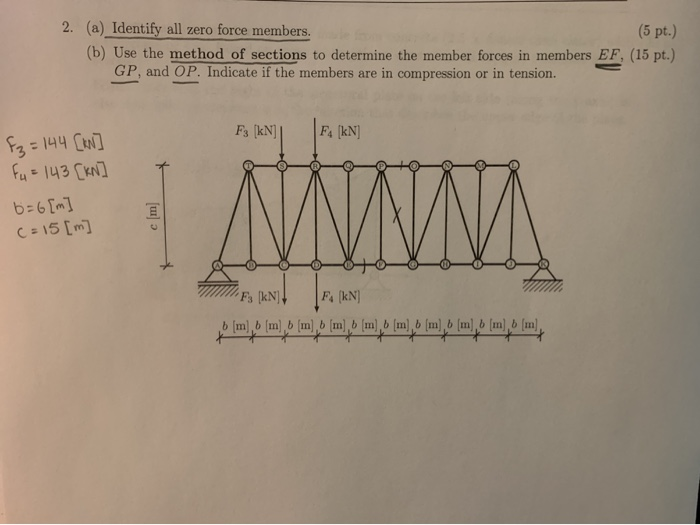a) Identify all zero force members b) Use the METHOD OF SECTIONS to determine the member forces in menbers EF, GP, OP. Indicate if the members are in compression or in tension. 2. (a) Identify all zero force members. (5 pt.) (b) Use the method of sections to determine the member forces in members EF, (15 pt.) GP, and OP. Indicate if the members are in compression or in tension. F3 [kN] Fs [kN] = 144 [kW] Fu = 143...

• For the roof truss shown, use method of sections to determine the forces in members BC and EF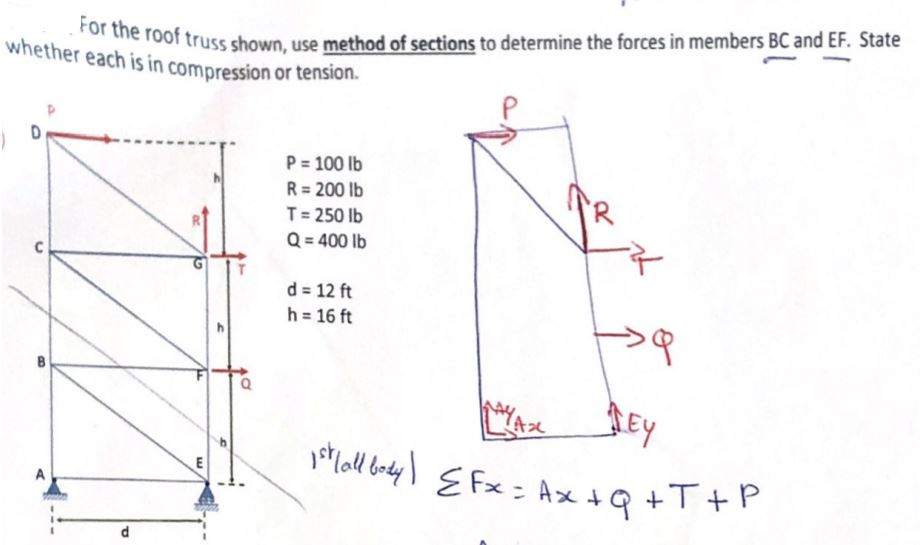For the roof truss shown, use method of sections to determine the forces in members BC and EF. State whether each is in compression or tension.